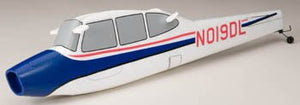# Great Planes Fuselage Set Flylite Ep Arf Gpma2582

Vendor
Great Planes
Regular price
Sold out
Sale price
\$64.18
Quantity must be 1 or more

• T

• h

• i

• s

• i

• s

• t

• h

• e

• G

• r

• e

• a

• t

• P

• l

• a

• n

• e

• s

• F

• u

• s

• e

• l

• a

• g

• e

• S

• e

• t

• f

• o

• r

• t

• h

• e

• E

• l

• e

• c

• t

• r

• i

• F

• l

• y

• F

• l

• y

• l

• i

• t

• e

• E

• P

• A

• R

• F

• A

• i

• r

• p

• l

• a

• n

• e

• .

• F

• e

• a

• t

• u

• r

• e

• s

• :

• W

• h

• i

• t

• e

• a

• n

• d

• b

• l

• u

• e

• c

• o

• l

• o

• r

• e

• d

• A

• e

• r

• o

• C

• e

• l

• l

• f

• o

• a

• m

• c

• o

• n

• s

• t

• r

• u

• c

• t

• i

• o

• n

• F

• o

• r

• t

• h

• e

• G

• r

• e

• a

• t

• P

• l

• a

• n

• e

• s

• E

• l

• e

• c

• t

• r

• i

• F

• l

• y

• F

• l

• y

• l

• i

• t

• e

• E

• P

• A

• R

• F

• A

• i

• r

• p

• l

• a

• n

• e

• P

• r

• e

• i

• n

• s

• t

• a

• l

• l

• e

• d

• t

• a

• i

• l

• w

• h

• e

• e

• l

• a

• s

• s

• e

• m

• b

• l

• y

• ,

• p

• u

• s

• h

• r

• o

• d

• s

• ,

• w

• o

• o

• d

• e

• n

• s

• e

• r

• v

• o

• t

• r

• a

• y

• s

• ,

• w

• i

• n

• g

• d

• o

• w

• e

• l

• s

• a

• n

• d

• b

• a

• t

• t

• e

• r

• y

• h

• a

• t

• c

• h

• I

• n

• c

• l

• u

• d

• e

• s

• :

• O

• n

• e

• G

• r

• e

• a

• t

• P

• l

• a

• n

• e

• s

• F

• l

• y

• l

• i

• t

• e

• F

• u

• s

• e

• l

• a

• g

• e

• R

• e

• q

• u

• i

• r

• e

• s

• :

• I

• n

• s

• t

• a

• l

• l

• i

• n

• g

• a

• l

• l

• c

• o

• m

• p

• o

• n

• e

• n

• t

• s

• i

• n

• t

• o

• a

• n

• d

• o

• n

• t

• o

• f

• u

• s

• e

• l

• a

• g

• e

This is the Great Planes Fuselage Set for the ElectriFly Flylite EP ARF Airplane.

Features:

• White and blue colored
• AeroCell foam construction
• For the Great Planes ElectriFly Flylite EP ARF Airplane
• Preinstalled tailwheel assembly, pushrods, wooden servo trays, wing dowels and battery hatch

Includes:
One Great Planes Flylite Fuselage

Requires:
Installing all components into and onto fuselage